## Two long, parallel wires are separated by a distance of 3.80 cm . The force per unit length that each wire exerts on the other is 4.90×10−5

Question

Two long, parallel wires are separated by a distance of 3.80 cm . The force per unit length that each wire exerts on the other is 4.90×10−5 N/m , and the wires repel each other. The current in one wire is 0.540 A .(a) What is the current in the second wire? (b) Are the two currents in the same direction or in opposite directions?

in progress 0
2 months 2021-07-29T05:19:30+00:00 1 Answers 5 views 0

(a). I’ = 17.24 A

(b).The current in second wire is in opposite direction compare to first wire.

Explanation:

Given data

r = 0.038 m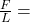4.9 ×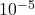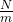I = 0.54 A

(a). The current in the second wire is calculated as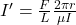Put all the values in above formula we get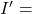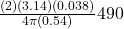I’ = 17.24 A

(b). The current in the two wires is in opposite directions because the wires repeal each other. therefore the current in second wire is in opposite direction compare to first wire.